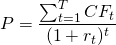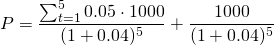# Fixed rate bond valuation

Fixed rate bond valuation literally forms the basis for a lot of other asset valuation models and is based on the method for discounting cash flows.

## Fixed rate bond valuation ingredients

Determining the value of a bond relies on determining the future cash flows and the appropriate discount rates. What the future cash flows will look like, in turn depends on the bond’s maturity, the principal, and the coupon rate. The following formula demonstrates how a fixed rate bond can be valuedFor example, consider a bond with a face value of 1000, 5 years to maturity and an annual coupon rate of 5% (paid on an annual basis). The future cash flows in year 1 to year 4 will then be 5% of 1000: 50. The cash flow in the fifth year will be equal to 1050 since the principal is then also repaid. Suppose the market discount rate is 4% a year (in every year). The value of the bond will then equal 1044.52, which is higher than the face value of a 1000. This is because the market discount rate today (4%) is lower than the coupon rate (5%) received on the bond. Therefore, the value of the bond goes up such that an investor who wants to buy this bond will only have a (effective rate of) return of 4%.## Bond valuation and the yield curve

Of course in real life, most of the time it is not the case the market discount rate is the same for all maturities. Most of the time, the so-called yield curve is upward rising. This means that the annual market interest rate for 1 year may be 1%, while the annual market interest rate for 2 years may be 3%, for 3 years 4% and so on. In that case, the interest rate that is plugged in the denominator will vary year from year.

## Summary

Bond valuation forms the basis for a lot of other valuation models. To value a fix rate bond, we need to know the maturity, the principal, the coupon rate, and market discount rates. Additionally, the yield curve plays an important role when valueing bonds.

### Fixed rate bond valuation

Need to have more insights? Download our free excel file: fixed rate bond valuation.